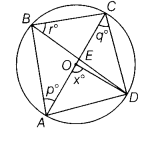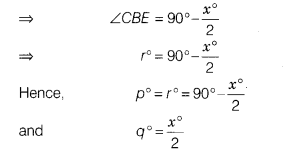# In the given figure, AC is the diameter of a circle with centre O and chord BD intersect

In the given figure, AC is the diameter of a circle with centre O and chord BD intersect AC at 90° at point E. Find the values of p, q, r in terms of x.Since, angle subtends by the arc at centre of circle is double the angle subtend it at remaining of the circle.